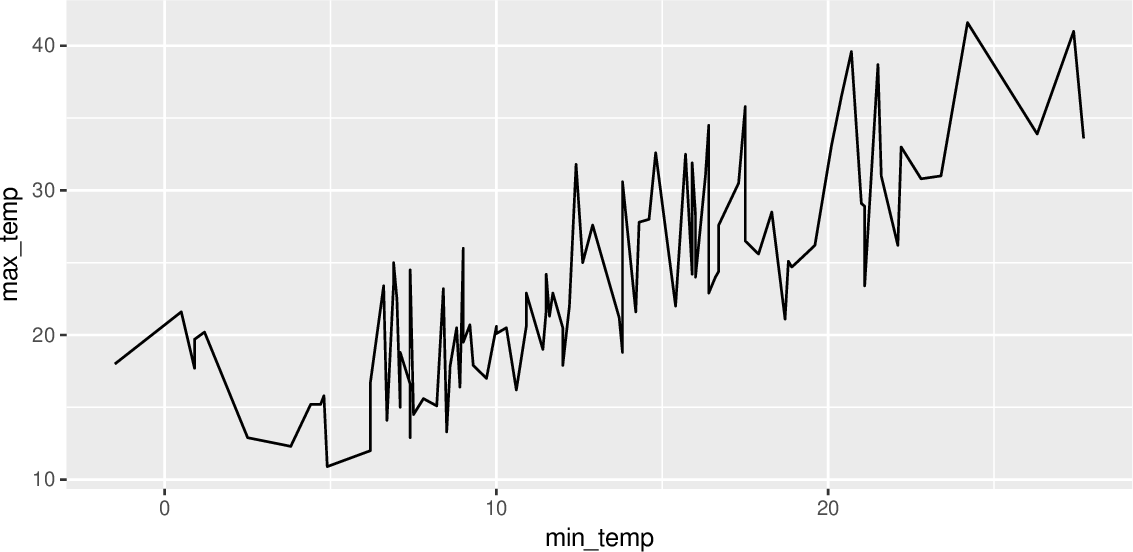Data Science Desktop Survival Guide by Graham WilliamsDesktop Survival Project Home Preface Data Science Introducing R R Constructs R Tasks R Strings R Read, Write, and Create Data Template Data Exploration Data Wrangling Data Visualisation Statistics ML Template ML Scenarios ML Activities ML Applications ML Algorithms Cluster Analysis Decision Trees Computer Vision Graph Data Privacy Literate Data Science Coding with Style Resources Bibliography Index

## Line Chart Basic

20180603set.seed(26439) ds %>%   sample_n(100) %>%   ggplot(aes(x=min_temp, y=max_temp)) +   geom_line() As an alternative to plotting the points we could join the dots and plot a line. One reason for doing so might be to attempt to observe more clearly any relationship between the two dimensions (i.e., the two variables we are plotting). Add a ggplot2::geom_line() to the canvas we can draw a line from left to right the follows the points exactly. The result is a rather jugged line and it is debatable whether it has added any further insight into our understanding of the data.